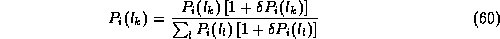Region Growing

Region growing approach is the opposite of the split and merge approach:
• An initial set of small areas are iteratively merged according to similarity constraints.
• Start by choosing an arbitrary seed pixel and compare it with neighbouring pixels (see Fig 37).
• Region is grown from the seed pixel by adding in neighbouring pixels that are similar, increasing the size of the region.
• When the growth of one region stops we simply choose another seed pixel which does not yet belong to any region and start again.

• This whole process is continued until all pixels belong to some region.
• A bottom up method.

Region growing methods often give very good segmentations that correspond well to the observed edges.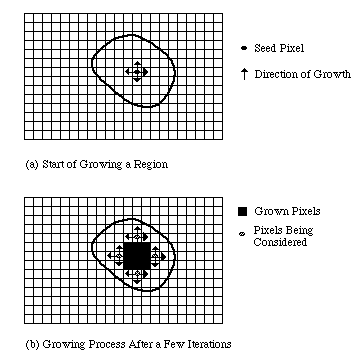Fig. 37 Example of region growing
However starting with a particular seed pixel and letting this region grow completely before trying other seeds biases the segmentation in favour of the regions which are segmented first.
This can have severalundesirable effects:
• Current region dominates the growth process -- ambiguities around edges of adjacent regions may not be resolved correctly.
• Different choices of seeds may give different segmentation results.
• Problems can occur if the (arbitrarily chosen) seed point lies on an edge.

To counter the above problems, simultaneous region growing techniques have been developed.
• Similarities of neighbouring regions are taken into account in the growing process.
• No single region is allowed to completely dominate the proceedings.
• A number of regions are allowed to grow at the same time.
• similar regions will gradually coalesce into expanding regions.
• Control of these methods may be quite complicated but efficient methods have been developed.
• Easy and efficient to implement on parallel computers.

Region Splitting

The basic idea of region splitting is to break the image into a set of disjoint regions which are coherent within themselves:
• Initially take the image as a whole to be the area of interest.
• Look at the area of interest and decide if all pixels contained in the region satisfy some similarity constraint.
• If TRUE then the area of interest corresponds to a region in the image.
• If FALSE split the area of interest (usually into four equal sub-areas) and consider each of the sub-areas as the area of interest in turn.

• This process continues until no further splitting occurs. In the worst case this happens when the areas are just one pixel in size.
• This is a divide and conquer or top down method.

If only a splitting schedule is used then the final segmentation would probably contain many neighbouring regions that have identical or similar properties.
Thus, a merging process is used after each split which compares adjacent regions and merges them if necessary. Algorithms of this nature are called split and merge algorithms.
To illustrate the basic principle of these methods let us consider an imaginary image.
• Letdenote the whole image shown in Fig 35(a).
• Not all the pixels inare similar so the region is split as in Fig 35(b).
• Assume that all pixels within regions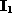,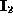andrespectively are similar but those in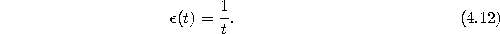are not.
• Thereforeis split next as in Fig 35(c).
• Now assume that all pixels within each region are similar with respect to that region, and that after comparing the split regions, regions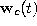and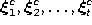are found to be identical.
• These are thus merged together as in Fig 35(d).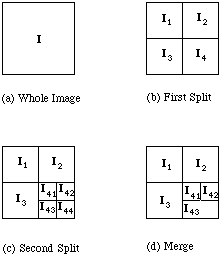Fig. 35 Example of region splitting and merging
We can describe the splitting of the image using a tree structure, using a modified quadtree. Each non-terminal node in the tree has at most four descendants, although it may have less due to merging. See Fig. 36.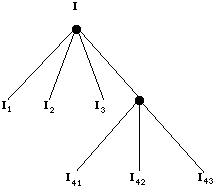Fig. 36 Region splitting and merging tree

Relaxation Labelling Methods

• Have already seen some applications of relaxation labelling
• Relaxation labelling for model based matching is no different.
• Matching is posed as a labelling problem, where a model primitive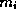is labelled with (i.e. matched to) a scene primitive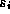.
• Region based measurements of both a numerical and topological nature used.
• Each primitive is given a quality measurement, normally a probability, for the likelihood of it labelling each model primitive.
• Starting from these initial measurements, the goal of the relaxation technique is to reduce iteratively the ambiguity and disagreement of the initial labels by employing a coherence measure for the set of matches.
• The compatibility of each primitive with its neighbourhood is used, and iteratively increasing the size of the neighbourhood.
• Relaxation labelling problems may be easily visualised as graph labelling problems.

Relaxation Labelling

Relaxation labelling techniques can be applied to many areas of computer vision.
Relaxation techniques have been applied to many other areas of computation in general, particularly to the solution of simultaneous nonlinear equations.
We shall first describe the basic principles behind relaxation labelling methods and then discuss various applications.
The basic elements of the relaxation labelling method are a set of features belonging to an object and a set of labels.
In the context of vision, these features are usually points, edges and surfaces.
Normally, the labelling schemes used are probabilistic in that for each feature, weights or probabilities are assigned to each label in the set giving an estimate of the likelihood that the particular label is the correct one for that feature.
Probabilistic approaches are then used to maximise (or minimise) the probabilities by iterative adjustment, taking into account the probabilities associated with neighbouring features.
Relaxation strategies do not necessarily guarantee convergence, and thus, we may not arrive at a final labelling solution with a unique label having probability one for each feature.
Let us now consider the labelling approach in more detail. Let us assume:
• O is the set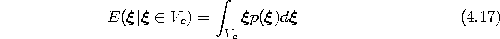of n object features to be labelled.
• L is the set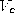of m possible labels for the features.

Let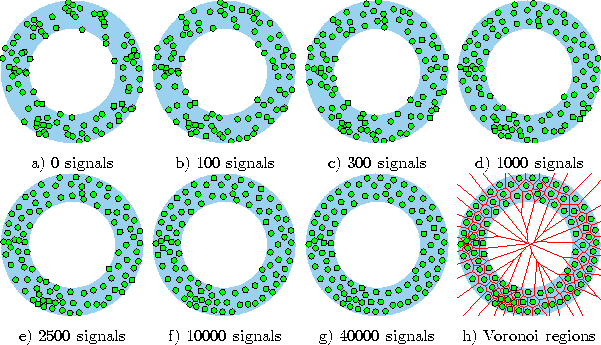be the probability that the label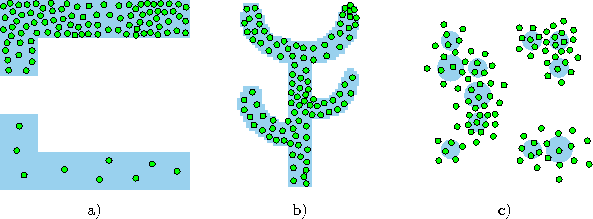is the correct label for object feature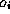.
The usual probability axioms can be applied that:
• Each probability satisfies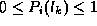where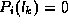implies that labelis impossible for featureand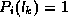implies that this labelling is certain.
• The set of labels are mutually exclusive and exhaustive. Thus we may write for each i: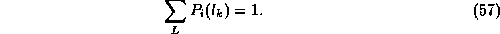Thus each feature is correctly described by exactly one label from the set of labels.

The labelling process starts with an initial, and perhaps arbitrary, assignment of probabilities for each label for each feature.
The basic algorithm then transforms these probabilities into to a new set according to some relaxation schedule.
This process is repeated until the labelling method converges or stabilises.
This occurs when little or no change occurs between successive sets of probability values.
Popular methods often take stochastic approaches to update the probability functions.
Here an operator considers the compatibility of label probabilities as constraints in the labelling algorithm.
The compatibility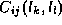is a correlation between labels defined as the conditional probability that featurehas a labelgiven that feature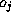has a label, i.e.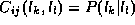.
Thus, updating the probabilities of labels is done by considering the probabilities of labels for neighbouring features.
Let us assume that we have changed all probabilities up to some step, S, and we now seek an updated probability for the next step S+1.
We can estimate the change in confidence ofby: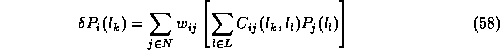where N is the set of features neighbouring, and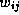is a factor that weights the labellings of these neighbours, defined in such a way that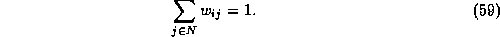The new probability for labelin generation S+1 can be computed from the values from generation S using